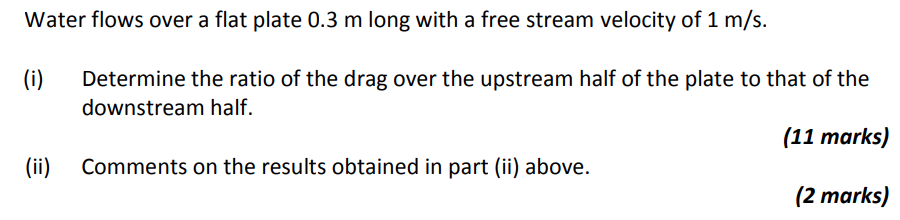Home / Expert Answers / Mechanical Engineering / water-flows-over-a-flat-plate-0-3-mathrm-m-long-with-a-free-stream-velocity-of-1-math-pa244

# (Solved): Water flows over a flat plate $$0.3 \mathrm{~m}$$ long with a free stream velocity of $$1 \math ...Water flows over a flat plate \( 0.3 \mathrm{~m}$$ long with a free stream velocity of $$1 \mathrm{~m} / \mathrm{s}$$. (i) Determine the ratio of the drag over the upstream half of the plate to that of the downstream half. (11 marks) (ii) Comments on the results obtained in part (ii) above. (2 marks)

We have an Answer from Expert